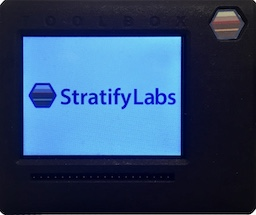###### Introducing the Stratify Toolbox

Are you a printf()-er or a code-stepper? The Stratify Toolbox is a printf()-ing awesome debug tool!

Microcontroller pins can usually output 3 states: low, high, and floating (aka high-impedance). However, microcontroller inputs don’t automatically measure these three states. For inputs, you can configure the pin as pullup, pulldown, or floating. With a little firmware, you can measure if an incoming pin is high, low, or floating.

The technique is:

• Pull the input high and measure
• Pull the input low and measure
• If high is high and low is low, the pin is floating
• If high is low and low is low, the pin is low
• If high is high and low is high, the pin is high

Here is the code using the `hal::Pin object` from the StratifyAPI.

``````#include <sapi/hal.hpp>

bool is_floating(Pin & input){
bool low;
bool high;

input.set_input(Pin::IS_PULLUP);
//you may have to insert a short delay so the signal can settle
high = input.get_value();
input.set_input(Pin::IS_PULLDOWN);
//you may have to insert a short delay so the signal can settle
low = input.get_value();
input.set_input(Pin::IS_FLOAT); //leave in floating state
return (high != low);
}
``````

That’s it. If you have two microcontrollers, you can use the floating state as another way to pass data back and forth. For example, if you are using an acknowledge line it can be: